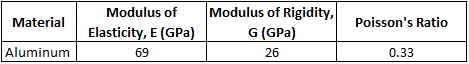## Bulk Modulus of Elasticity

Consider a 3 m long extrusion with a square shaped cross sectional area of 0.25 m^2 is made from Aluminum. What is the bulk modulus of elasticity in GPa? Note the properties of Aluminum in the table shown.Hint
A substance’s bulk modulus describes how resistant the material is to compression.
Hint 2
Bulk (Volume) Modulus of Elasticity:
$$K=\frac{E}{3(1-2\nu )}$$$where $$K$$ is the bulk modulus, $$E$$ is the modulus of elasticity, and $$\nu$$ is Poisson’s ratio. A substance’s bulk modulus describes how resistant the material is to compression. Bulk (Volume) Modulus of Elasticity: $$K=\frac{E}{3(1-2\nu )}$$$
where $$K$$ is the bulk modulus, $$E$$ is the modulus of elasticity, and $$\nu$$ is Poisson’s ratio.

Notice how the extrusion dimensions, cross sectional area, and Modulus of Rigidity values can be ignored. They were in the problem statement to cause confusion. Plugging in the modulus of elasticity and Poisson’s ratio values from the Aluminum properties table:
$$K=\frac{69GPa}{3[1-2(0.33)]}=\frac{69GPa}{3[1-0.66]}=\frac{69GPa}{3(0.34)}=\frac{69GPa}{1.02}=67.6\:GPa$$\$
67.6 GPa